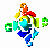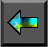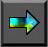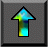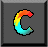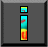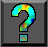Next: Chapter Notes Up: 3 A Quantitative Basis for Design Previous: 3.10 Summary

# Exercises

The exercises in this chapter are designed to provide experience in the development and use of performance models. When an exercise asks you to implement an algorithm, you should use one of the programming tools described in Part II.

1. Discuss the relative importance of the various performance metrics listed in Section 3.1 when designing a parallel floorplan optimization program for use in VLSI design.

2. Discuss the relative importance of the various performance metrics listed in Section 3.1 when designing a video server that uses a parallel computer to generate many hundreds of thousands of concurrent video streams. Each stream must be retrieved from disk, decoded, and output over a network.

3.   The self-consistent field (SCF) method in computational chemistry   involves two operations: Fock matrix construction and matrix   diagonalization. Assuming that diagonalization accounts for 0.5 per cent of total execution time on a uniprocessor computer, use Amdahl's law to determine the maximum speedup that can be obtained if only the Fock matrix construction operation is parallelized.

4. You are charged with designing a parallel SCF program. You estimate your Fock matrix construction algorithm to be 90 percent efficient on your target computer. You must choose between two parallel diagonalization algorithms, which on five hundred processors achieve speedups of 50 and 10, respectively. What overall efficiency do you expect to achieve with these two algorithms? If your time is as valuable as the computer's, and you expect the more efficient algorithm to take one hundred hours longer to program, for how many hours must you plan to use the parallel program if the more efficient algorithm is to be worthwhile?

5. Some people argue that in the future, processors will become essentially free as the cost of computers become dominated by the cost of storage and communication networks. Discuss how this situation may affect algorithm design and performance analysis.

6. Generate an execution profile similar to that in Figure 3.8 for an implementation of a parallel finite difference algorithm based on a 2-D decomposition. Under which circumstances will message startups contribute more to execution time than will data transfer costs?

7.   Derive expressions that indicate when a 2-D decomposition of a finite difference computation on an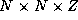grid will be superior to a 1-D decomposition and when a 3-D decomposition will be superior to a 2-D decomposition. Are these conditions likely to apply in practice? Let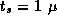sec,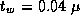sec,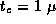sec, and P=1000 . For what values of N does the use of a 3-D decomposition rather than a 2-D decomposition reduce execution time by more than 10 percent?

8.   Adapt the analysis of Example 3.4 to consider 1-D and 2-D decompositions of a 2-D grid. Let N=1024 , and fix other parameters as in Exercise 7. For what values of P does the use of a 2-D decomposition rather than a 1-D decomposition reduce execution time by more than 10 percent?

9.   Implement a simple ``ping-pong'' program that bounces messages between a pair of processors. Measure performance as a function of message size on a workstation network and on one or more parallel computers. Fit your results to Equation 3.1 to obtain values for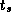and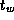. Discuss the quality of your results and of the fit.

10.   Develop a performance model for the program constructed in Exercise 5 in Chapter 2 that gives execution time as a function of N , P ,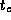,, and. Perform empirical studies to determine values for,, andon different parallel computer systems. Use the results of these studies to evaluate the adequacy of your model.

11. Develop performance models for the parallel algorithms developed in Exercise 10 in Chapter 2. Compare these models with performance data obtained from implementations of these algorithms.

12. Determine the isoefficiency function for the program developed in Exercise 10. Verify this experimentally.

13. Use the ``ping-pong'' program of Exercise 9 to study the impact of bandwidth limitations on performance, by writing a program in which several pairs of processors perform exchanges concurrently. Measure execution times on a workstation network and on one or more parallel computers. Relate observed performance to Equation 3.10.

14. Implement the parallel summation algorithm of Section 2.4.1. Measure execution times as a function of problem size on a network of workstations and on one or more parallel computers. Relate observed performance to the performance models developed in this chapter.

15. Determine the isoefficiency function for the butterfly summation algorithm of Section 2.4.1, with and without bandwidth limitations.

16. Design a communication structure for the algorithm Floyd 2 discussed in Section 3.9.1.

17.   Assume that a cyclic mapping is used in the atmosphere model of Section 2.6 to compensate for load imbalances. Develop an analytic expression for the additional communication cost associated with various block sizes and hence for the load imbalance that must exist for this approach to be worthwhile.

18. Implement a two-dimensional finite difference algorithm using a nine-point stencil. Use this program to verify experimentally the analysis of Exercise 17. Simulate load imbalance by calling a ``work'' function that performs different amounts of computation at different grid points.

19.   Assume that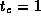,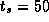, and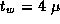sec. Use the performance models summarized in Table 3.7 to determine the values of N and P for which the various shortest-path algorithms of Section 3.9 are optimal.

20. Assume that a graph represented by an adjacency matrix of size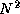is distributed among P tasks prior to the execution of the all-pairs shortest-path algorithm. Repeat the analysis of Exercise 19 but allow for the cost of data replication in the Dijkstra algorithms.

21. Extend the performance models developed for the shortest-path algorithms to take into account bandwidth limitations on a 1-D mesh architecture.

22. Implement algorithms Floyd 1 and Floyd 2, and compare their performance with that predicted by Equations 3.12 and 3.13. Account for any differences.

23. In so-called nondirect Fock matrix construction algorithms, the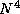integrals of Equation 2.3 are cached on disk and reused at each step. Discuss the performance issues that may arise when developing a code of this sort.

24. The bisection width of a computer is the minimum number of   wires that must be cut to divide the computer into two equal parts. Multiplying this by the channel bandwidth gives the bisection   bandwidth. For example, the bisection bandwidth of a 1-D mesh with bidirectional connections is 2/. Determine the bisection bandwidth of a bus, 2-D mesh, 3-D mesh, and hypercube.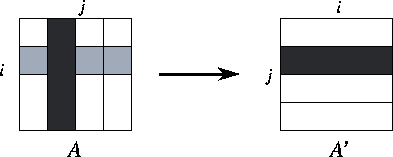Figure 3.29: Parallel matrix transpose of a matrix A decomposed by column, with P=4 . The components of the matrix allocated to a single task are shaded black, and the components required from other tasks are stippled.

25.   An array transpose operation reorganizes an array partitioned in one   dimension so that it is partitioned in the second dimension   (Figure 3.29). This can be achieved in P-1 steps, with each processor exchanging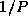of its data with another processor in each step. Develop a performance model for this operation.

26. Equation 3.1 can be extended to account for the distance D between originating and destination processors: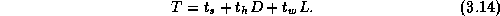The time per hop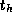typically has magnitude comparable to.   Under what circumstances might theterm be significant?

27. Develop a performance model for the matrix transpose algorithm on a 1-D mesh that takes into account per-hop costs, as specified by Equation 3.14. Assume that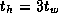and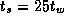, and identify P and N values for which per-hop costs make a significant ( >5 percent) difference to execution time.

28.   Demonstrate that the transpose algorithm's messages travel a total of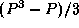hops on a 1-D mesh. Use this information to refine the performance model of Exercise 25 to account for competition for bandwidth.

29. In the array transpose algorithm of Exercise 25, roughly half of the array must be moved from one half of the computer to the other. Hence, we can obtain a lower time bound by dividing the data volume by the bisection bandwidth. Compare this bound with times predicted by simple and bandwidth-limited performance models, on a bus, one-dimensional mesh, and two-dimensional mesh.

30. Implement the array transpose algorithm and study its performance. Compare your results to the performance models developed in preceding exercises.Next: Chapter Notes Up: 3 A Quantitative Basis for Design Previous: 3.10 Summary